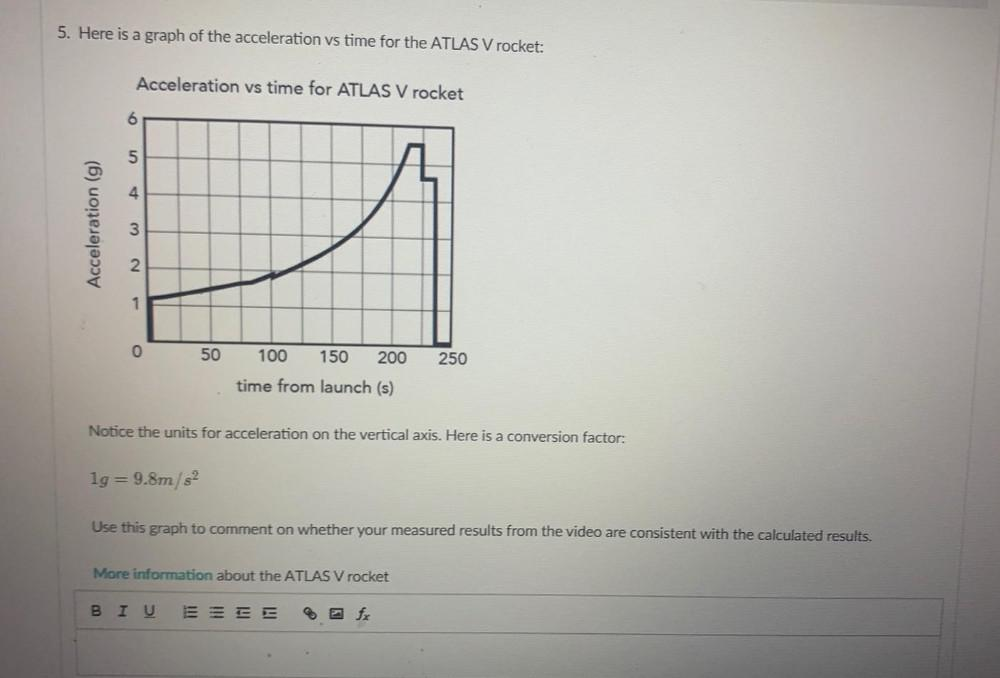Question:

# 5. Here is a graph of the acceleration vs time for the ATLAS V rocket: Acceleration vs time for ATLAS V rocket 6 5 4 Acceleratio5. Here is a graph of the acceleration vs time for the ATLAS V rocket: Acceleration vs time for ATLAS V rocket 6 5 4 Acceleration (g) 3 2 1 0 50 100 150 200 250 time from launch (s) Notice the units for acceleration on the vertical axis. Here is a conversion factor: lg = 9.8m/s2 Use this graph to comment on whether your measured results from the video are consistent with the calculated results. More information about the ATLAS V rocket BIU ES